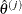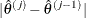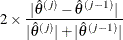### RANDOM Statement

RANDOM variable </ options> ;

The RANDOM statement enables you to fit a shared frailty model for clustered data (see the section The Frailty Model for details). The variable that represents the clusters must be a CLASS variable (declared in the CLASS statement).

The following options can be specified in the RANDOM statement:

ABSPCONV=r

specifies an absolute variance estimate convergence criterion for the doubly iterative estimation process. The PHREG procedure applies this criterion to the variance parameter estimate of the random effects. Supposedenotes the estimate of the variance parameter at the jth optimization. By default, PROC PHREG examines the relative change in the variance estimate between optimizations (see the PCONV= option). The purpose of the ABSPCONV= criterion is to stop the doubly iterative process when the absolute changeis less than the tolerance criterion r. This convergence criterion does not affect the convergence criteria applied within any individual optimization. In order to change the convergence behavior within an individual optimization, you can use the ABSCONV=, ABSFCONV=, ABSGCONV=, ABSXCONV=, FCONV=, or GCONV= option in the NLOPTIONS statement.

ALPHA=value

specifies thelevel of the confidence limits for the random effects. The default is the value of the ALPHA= option in the PROC PHREG statement, or 0.05 if that option is not specified. This option is ignored if the SOLUTION option is not also specified.

DIST=GAMMA | LOGNORMAL

specifies the distribution of the shared frailty. DIST=GAMMA specifies a gamma frailty model. DIST=LOGNORMAL specifies a lognormal frailty model; that is, the log-frailty random variable has a normal distribution with mean zero. The default is DIST=LOGNORMAL.

METHOD=REML | ML

specifies the estimation method for the variance parameter of the normal random effects. METHOD=REML performs the residual maximum likelihood; METHOD=ML performs maximum likelihood. This option is ignored for the gamma frailty model. The default is METHOD=REML.

NOCLPRINT

suppresses the display of the Class Level Information for Random Effects table.

PCONV=r

specifies the variance estimate convergence criterion for the doubly iterative estimation process. The PHREG procedure applies this criterion to the variance estimate of the random effects. Supposedenotes the estimate of variance at the jth optimization. The procedure terminates the doubly iterative process if the relative changeis less than r. To check an absolute convergence criterion in addition, you can specify the ABSPCONV= option in the RANDOM statement. The default value for r is 1E–4. This convergence criterion does not affect the convergence criteria applied within any individual optimization. In order to change the convergence behavior within an individual optimization, you can use the ABSCONV=, ABSFCONV=, ABSGCONV=, ABSXCONV=, FCONV=, or GCONV= option in the NLOPTIONS statement.

SOLUTION <(number-list)>

displays statistical measures of the random-effect parameters. The behavior of this option depends on whether you also specify the BAYES statement:

• When you do not specify a BAYES statement, this option displays point estimates and confidence intervals for the random components and for the frailties.

• When you also specify a BAYES statement, this option displays the summary statistics and diagnostics of the random-effect parameters. Optionally, you can specify a number-list of indices of the random-effect parameters for which the summary statistics and diagnostics are to be displayed. For example, to display the summary statistics and diagnostics for the second to the fifth random-effect parameters, specify

SOLUTION(2 to 5)


If you specify SOLUTION without a list, summary statistics and diagnostics are displayed for each random-effect parameter.

INITIALVARIANCE=value
INITIAL=value

specifies an initial value of the dispersion parameter. For the lognormal frailty model, the dispersion parameter represents the variance of the normal random effect; for the gamma frailty model, it represents the variance of the gamma frailty. The default is INITIAL=1.